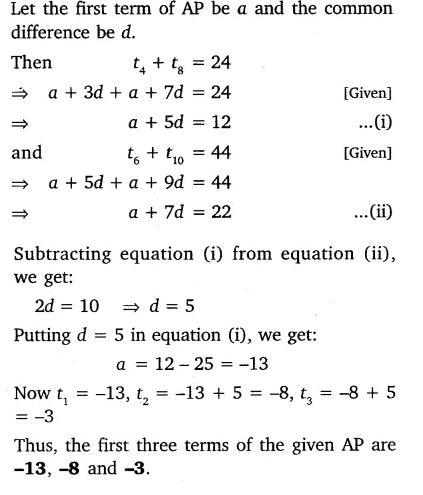# The sum of the 4th and 8th terms of an AP is 24 and the sum of the 6th and 10th terms is 44

The sum of the 4th and 8th terms of an AP is 24 and the sum of the 6th and 10th terms is 44. Find the first three terms of the AP.